# NCERT Solutions for Class 9 Science Chapter 10 – Gravitation

NCERT Solutions for Class 9 Science Chapter 10 – Gravitation, contains solutions to various questions in Exercises for Chapter 10. Gravitation Class 9 NCERT Solutions have been explained in a simple and easy to understand manner. We are providing all subjects which can be accessed by clicking here.

## Download NCERT Solutions for Class 9 Science Chapter 10 – Gravitation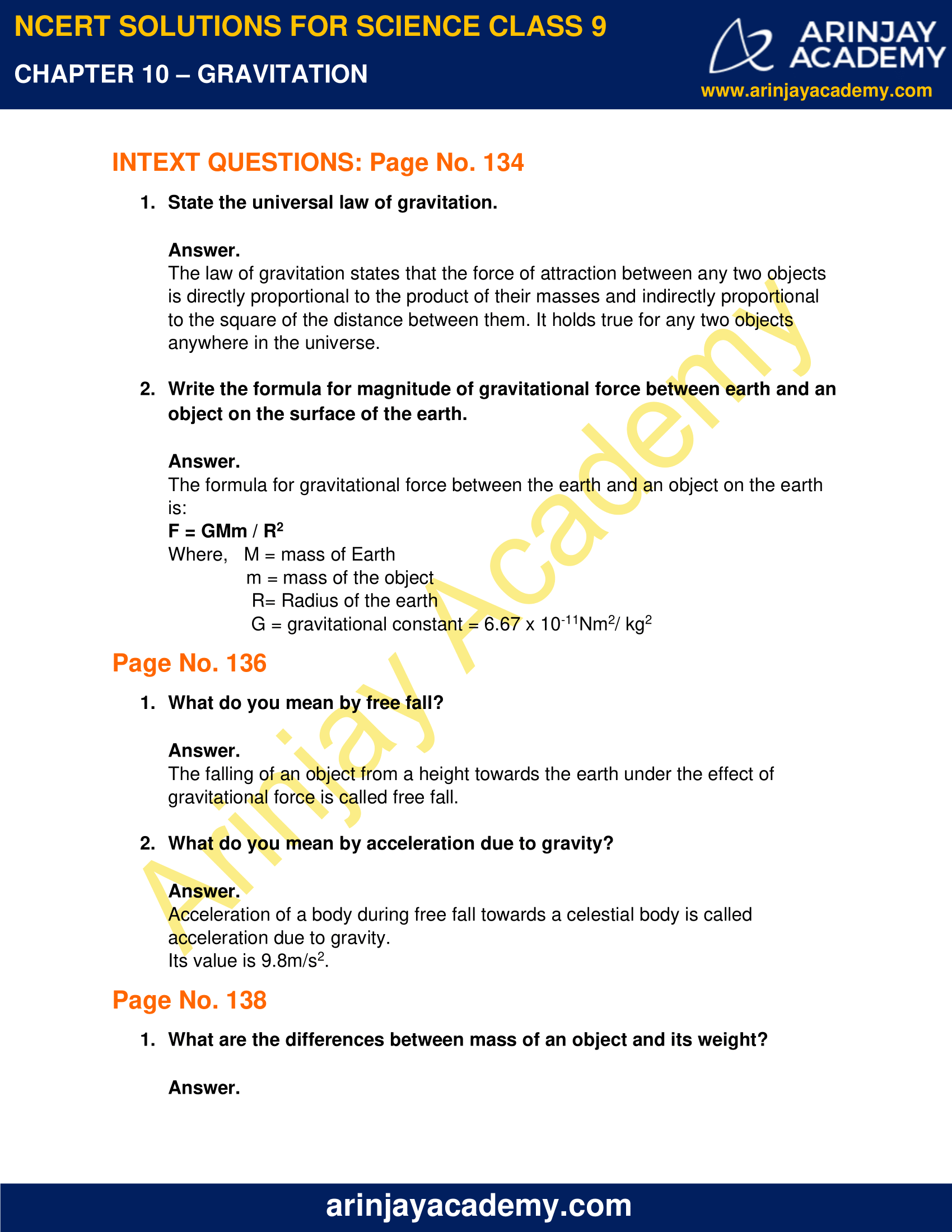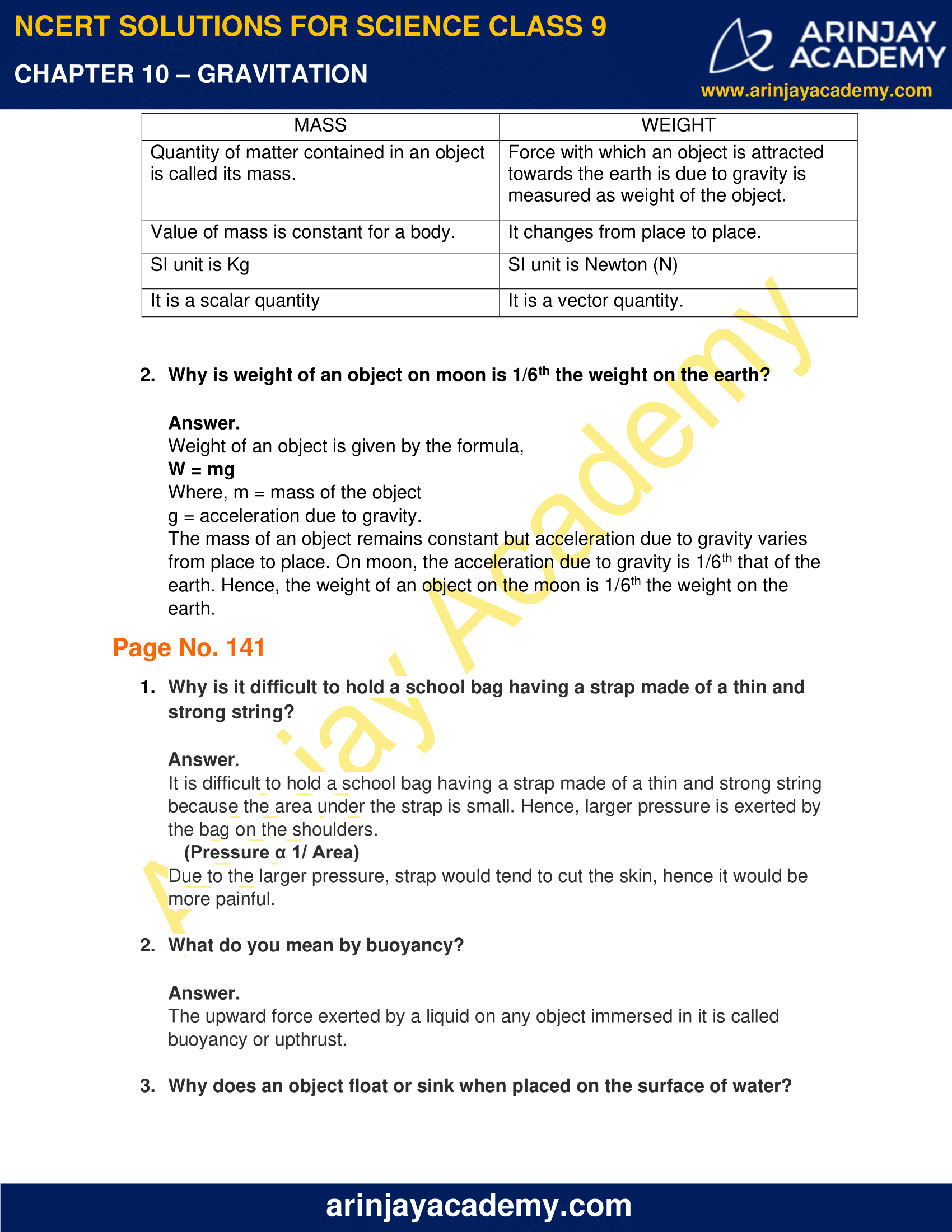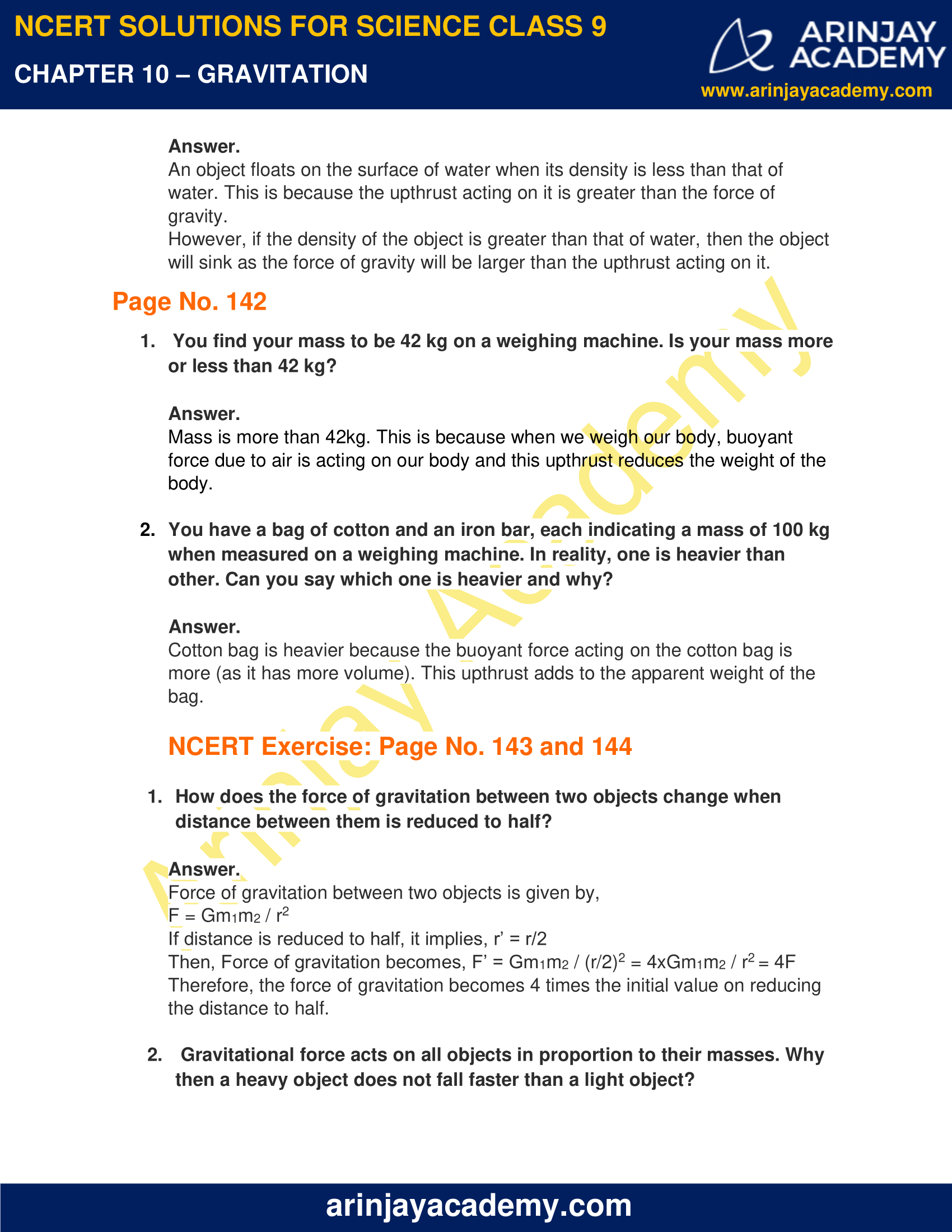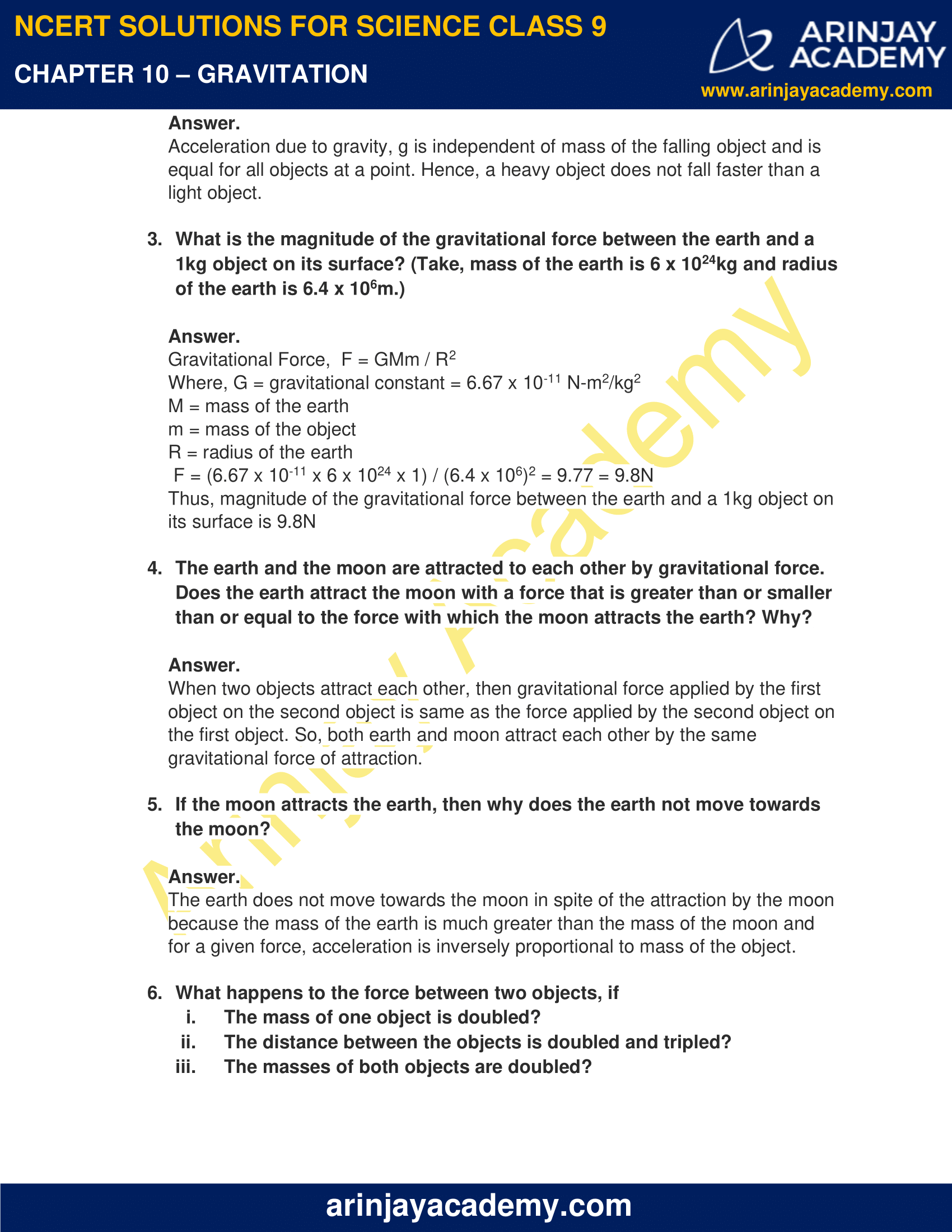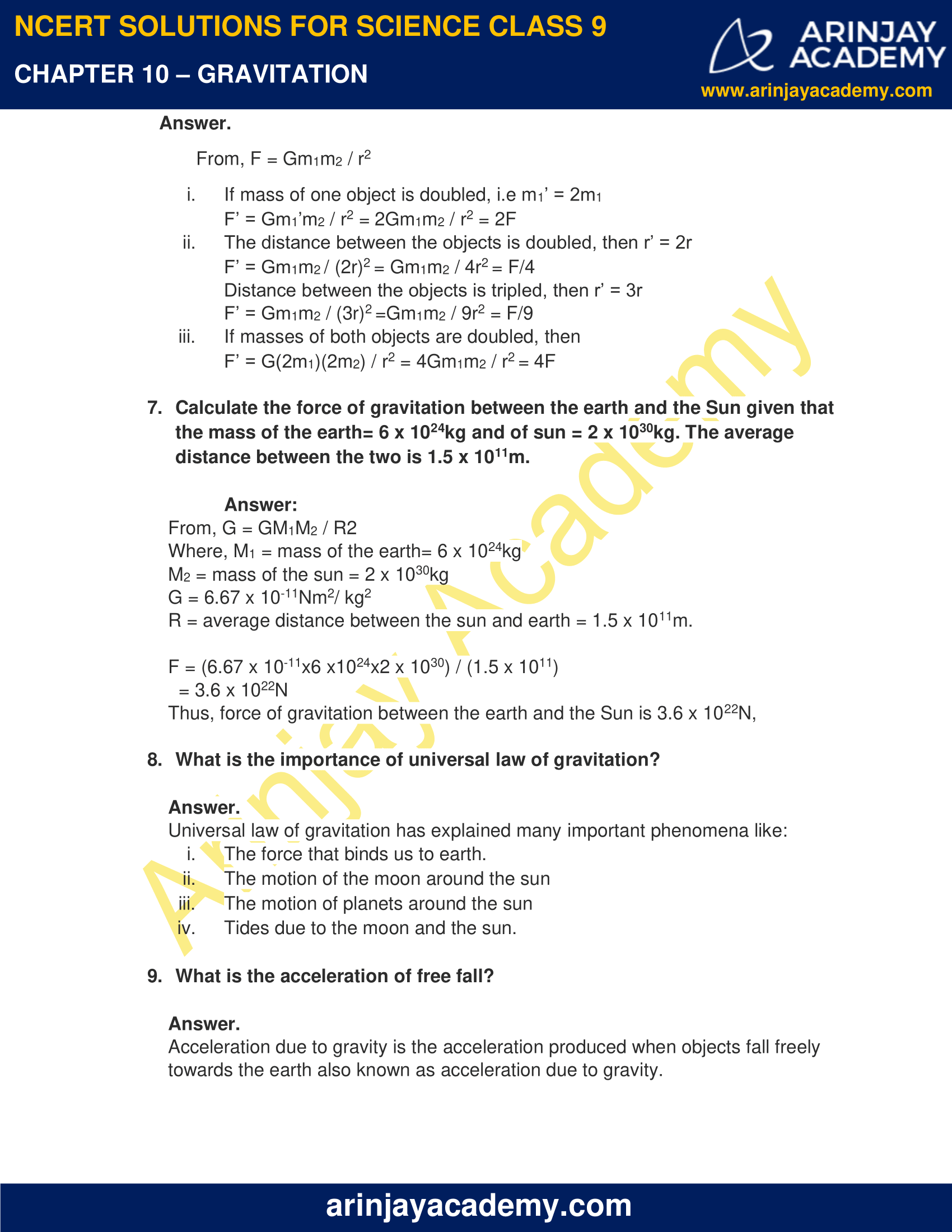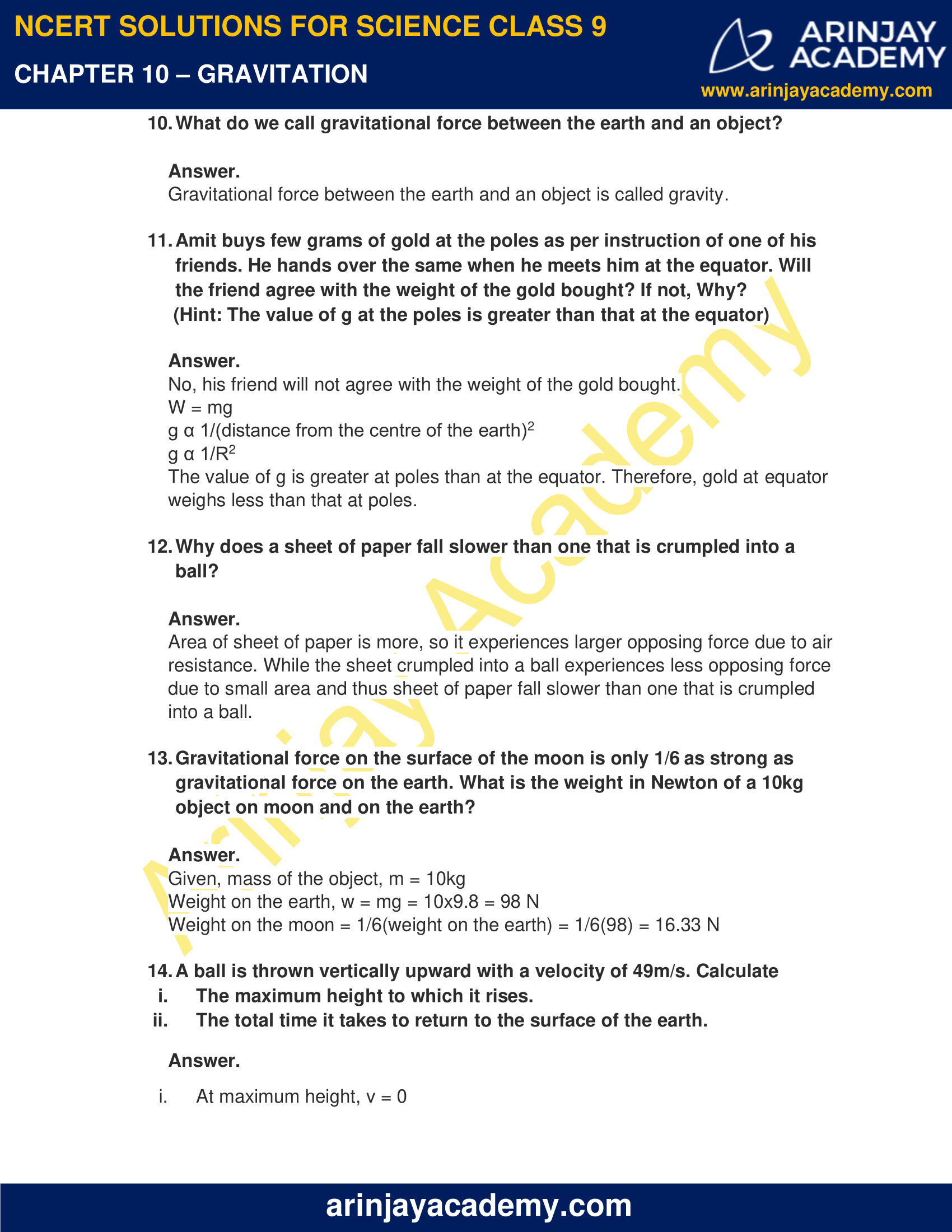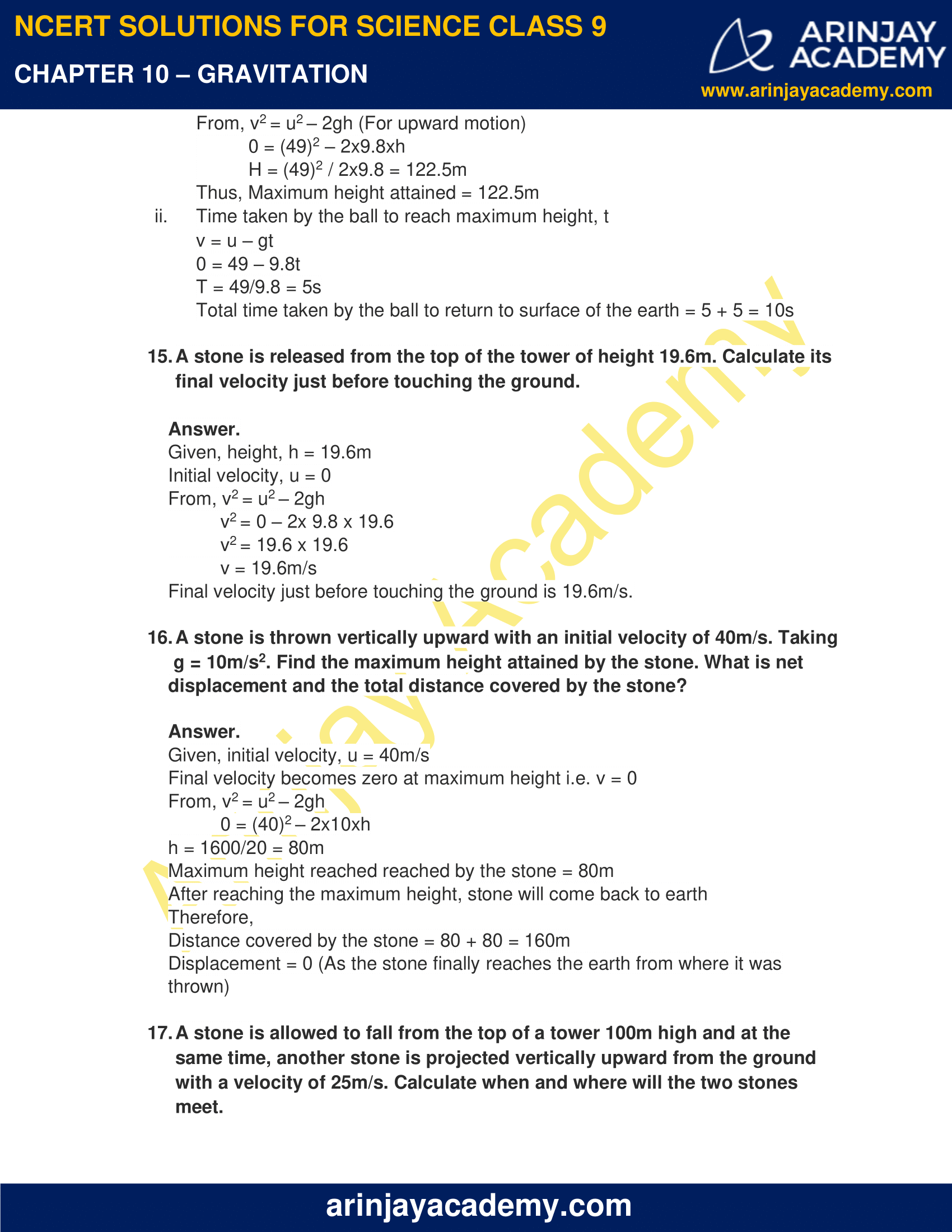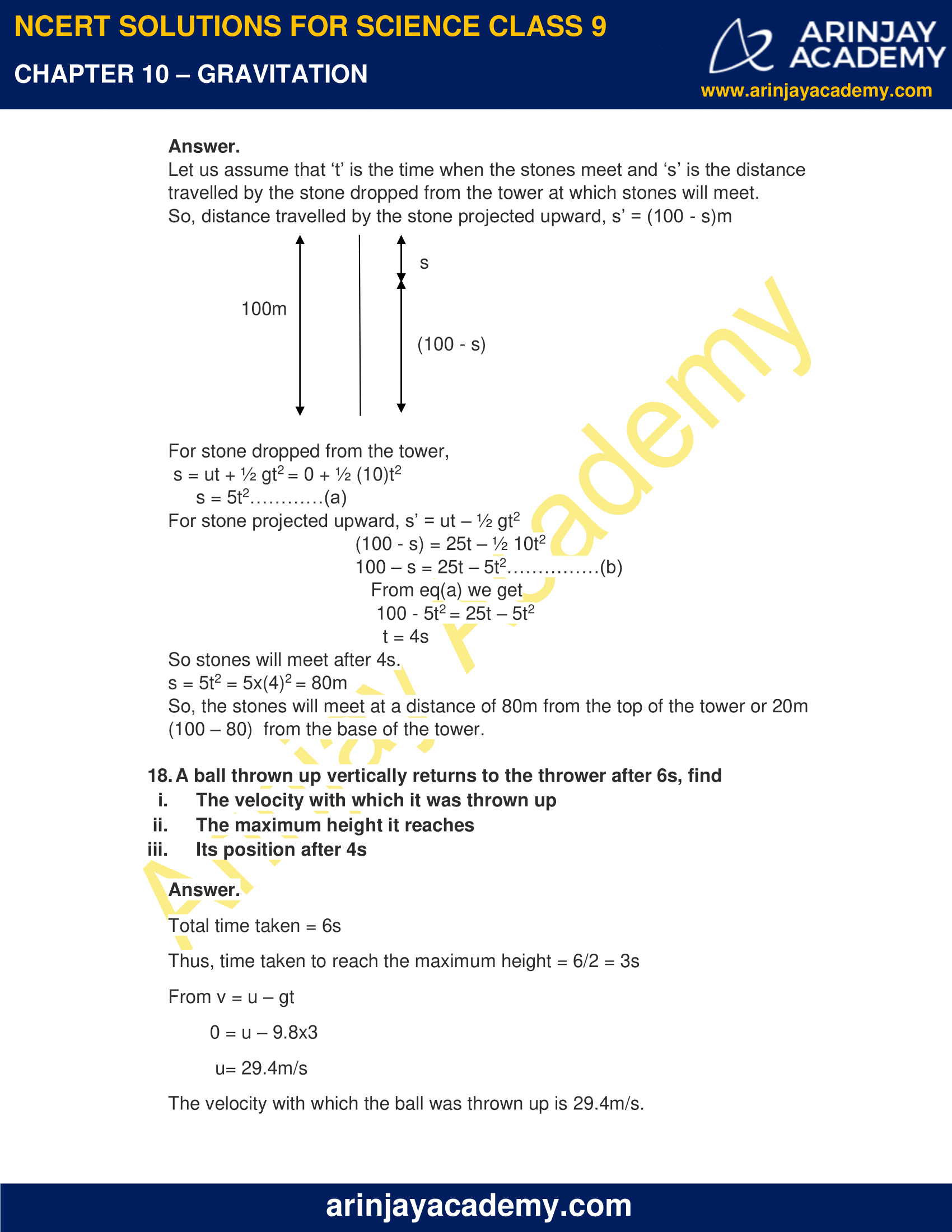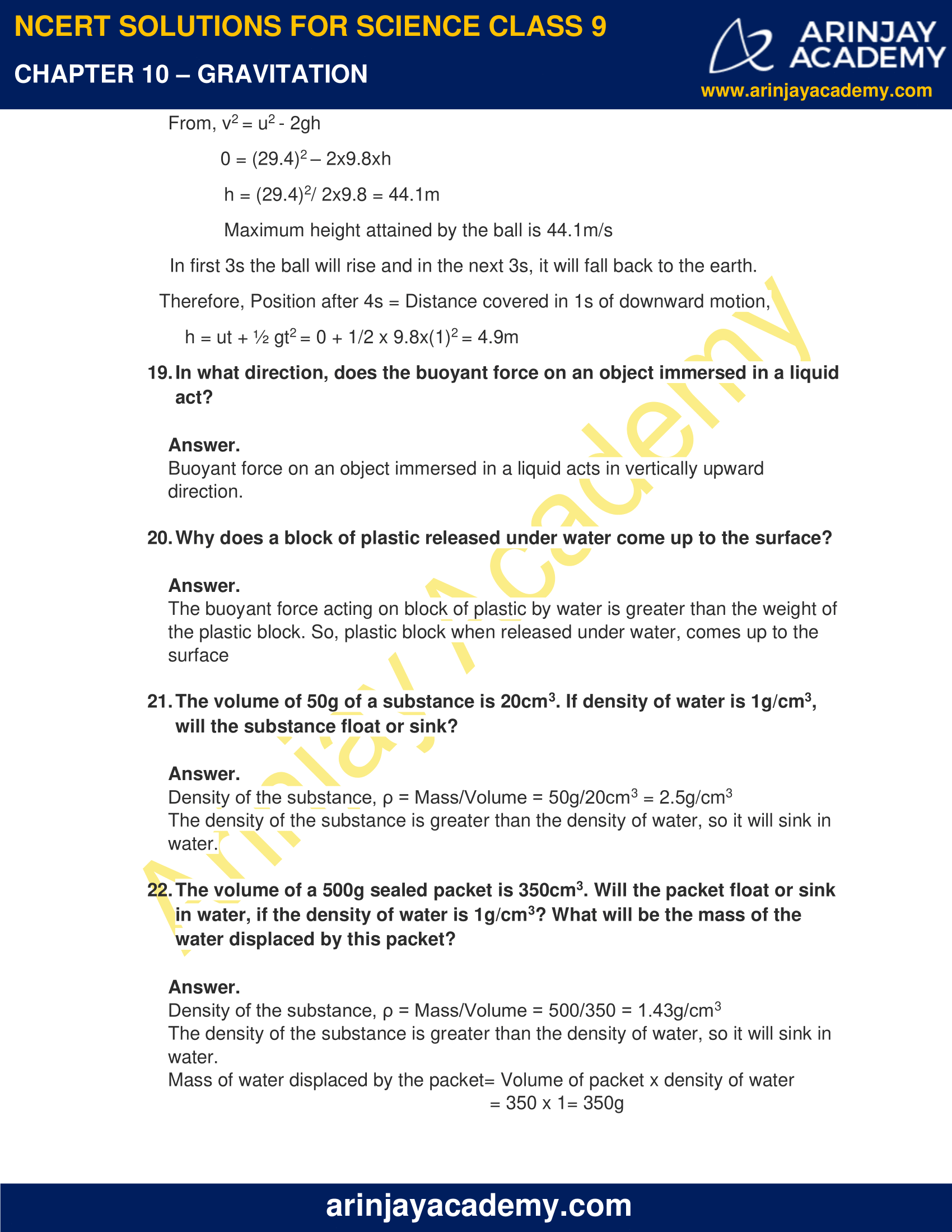### NCERT Solutions for Class 9 Science Chapter 10 – Gravitation – NCERT Exercises

NCERT Solutions for Class 9 Science Chapter 10 – Gravitation, Exercises includes all in text and exercise solved questions which helps you to understand the topic covered in Chapter 10 Gravitation, in a better manner to help you to score good marks in your examinations.

### NCERT Solutions for Class 9 Science Chapter 10 – Gravitation – Intext Questions – Page No. 134

1. State the universal law of gravitation.

The law of gravitation states that the force of attraction between any two objects is directly proportional to the product of their masses and indirectly proportional to the square of the distance between them. It holds true for any two objects anywhere in the universe.

2. Write the formula for magnitude of gravitational force between earth and an object on the surface of the earth.

The formula for gravitational force between the earth and an object on the earth is:

F = GMm / R2

Where,   M = mass of Earth

m = mass of the object

G = gravitational constant = 6.67 x 10-11Nm2/ kg2

### NCERT Solutions for Class 9 Science Chapter 10 – Gravitation – Intext Questions – Page No. 136

1. What do you mean by free fall?

The falling of an object from a height towards the earth under the effect of gravitational force is called free fall.

2. What do you mean by acceleration due to gravity?

Acceleration of a body during free fall towards a celestial body is called acceleration due to gravity.

Its value is 9.8m/s2.

Page No. 138

1. What are the differences between mass of an object and its weight?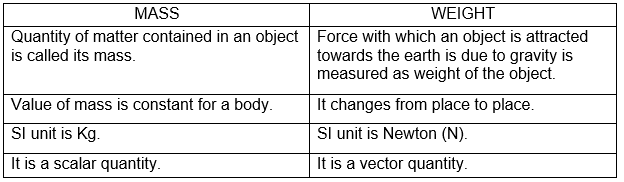2. Why is weight of an object on moon is 1/6th the weight on the earth?

Weight of an object is given by the formula,

W = mg

Where, m = mass of the object

g = acceleration due to gravity.

The mass of an object remains constant but acceleration due to gravity varies from place to place. On moon, the acceleration due to gravity is 1/6th that of the earth. Hence, the weight of an object on the moon is 1/6th the weight on the earth.

### NCERT Solutions for Class 9 Science Chapter 10 – Gravitation – Intext Questions – Page No. 141

1. Why is it difficult to hold a school bag having a strap made of a thin and strong string?

It is difficult to hold a school bag having a strap made of a thin and strong string because the area under the strap is small. Hence, larger pressure is exerted by the bag on the shoulders.

(Pressure α 1/ Area)

Due to the larger pressure, strap would tend to cut the skin, hence it would be more painful.

2. What do you mean by buoyancy?

The upward force exerted by a liquid on any object immersed in it is called buoyancy or upthrust.

3. Why does an object float or sink when placed on the surface of water?

An object floats on the surface of water when its density is less than that of water. This is because the upthrust acting on it is greater than the force of gravity.

However, if the density of the object is greater than that of water, then the object will sink as the force of gravity will be larger than the upthrust acting on it.

### Page No. 142

1. You find your mass to be 42 kg on a weighing machine. Is your mass more or less than 42 kg ?

Mass is more than 42 kg. This is because when we weigh our body, buoyant force due to air is acting on our body and this upthrust reduces the weight of the body.

2. You have a bag of cotton and an iron bar, each indicating a mass of 100 kg when measured on a weighing machine. In reality, one is heavier than other. Can you say which one is heavier and why?

Cotton bag is heavier because the buoyant force acting on the cotton bag is more (as it has more volume). This upthrust adds to the apparent weight of the bag.

### NCERT Solutions for Class 9 Science Chapter 10 – Gravitation – NCERT Exercise: Page No. 143 and 144

1. How does the force of gravitation between two objects change when distance between them is reduced to half?

Force of gravitation between two objects is given by,

F = Gm1m2 / r2

If distance is reduced to half, it implies, r’ = r/2

Then, Force of gravitation becomes, F’ = Gm1m2 / (r/2)2 = 4xGm1m2 / r2 = 4F

Therefore, the force of gravitation becomes 4 times the initial value on reducing the distance to half.

2. Gravitational force acts on all objects in proportion to their masses. Why then a heavy object does not fall faster than a light object?

Acceleration due to gravity, g is independent of mass of the falling object and is equal for all objects at a point. Hence, a heavy object does not fall faster than a light object.

3. What is the magnitude of the gravitational force between the earth and a 1kg object on its surface? (Take, mass of the earth is 6 x 1024kg and radius of the earth is 6.4 x 106)

Gravitational Force,  F = GMm / R2

Where, G = gravitational constant = 6.67 x 10-11 N-m2/kg2

M = mass of the earth

m = mass of the object

R = radius of the earth

F = (6.67 x 10-11 x 6 x 1024 x 1) / (6.4 x 106)2 = 9.77 = 9.8N

Thus, magnitude of the gravitational force between the earth and a 1kg object on its surface is 9.8N

4. The earth and the moon are attracted to each other by gravitational force. Does the earth attract the moon with a force that is greater than or smaller than or equal to the force with which the moon attracts the earth? Why?

When two objects attract each other, then gravitational force applied by the first object on the second object is same as the force applied by the second object on the first object. So, both earth and moon attract each other by the same gravitational force of attraction.

5. If the moon attracts the earth, then why does the earth not move towards the moon?

The earth does not move towards the moon in spite of the attraction by the moon because the mass of the earth is much greater than the mass of the moon and for a given force, acceleration is inversely proportional to mass of the object.

6. What happens to the force between two objects, if

i. The mass of one object is doubled?
ii. The distance between the objects is doubled and tripled?
iii. The masses of both objects are doubled?

From, F = Gm1m2 / r2

i. If mass of one object is doubled, i.e m1’ = 2m1

F’ = Gm1’m2 / r2 = 2Gm1m2 / r2 = 2F

ii. The distance between the objects is doubled, then r’ = 2r

F’ = Gm1m2 / (2r)2 = Gm1m2 / 4r2 = F/4

Distance between the objects is tripled, then r’ = 3r

F’ = Gm1m2 / (3r)2 =Gm1m2 / 9r2 = F/9

iii. If masses of both objects are doubled, then

F’ = G(2m1)(2m2) / r2 = 4Gm1m2 / r2 = 4F

7. Calculate the force of gravitation between the earth and the Sun given that the mass of the earth= 6 x 1024kg and of sun = 2 x 1030 The average distance between the two is 1.5 x 1011m.

From, G = GM1M2 / R2

Where, M1 = mass of the earth= 6 x 1024kg

M2 = mass of the sun = 2 x 1030kg

G = 6.67 x 10-11Nm2/ kg2

R = average distance between the sun and earth = 1.5 x 1011m.

F = (6.67 x 10-11x6 x1024x2 x 1030) / (1.5 x 1011)

= 3.6 x 1022N

Thus, force of gravitation between the earth and the Sun is 3.6 x 1022N,

8. What is the importance of universal law of gravitation?

Universal law of gravitation has explained many important phenomena like:

i. The force that binds us to earth.
ii. The motion of the moon around the sun
iii. The motion of planets around the sun
iv. Tides due to the moon and the sun.

9. What is the acceleration of free fall?

Acceleration due to gravity is the acceleration produced when objects fall freely towards the earth also known as acceleration due to gravity.

10. What do we call gravitational force between the earth and an object?

Gravitational force between the earth and an object is called gravity.

11. Amit buys few grams of gold at the poles as per instruction of one of his friends. He hands over the same when he meets him at the equator. Will the friend agree with the weight of the gold bought? If not, Why?

(Hint: The value of g at the poles is greater than that at the equator)

No, his friend will not agree with the weight of the gold bought.

W = mg

g α 1/(distance from the centre of the earth)2

g α 1/R2

The value of g is greater at poles than at the equator. Therefore, gold at equator weighs less than that at poles.

12. Why does a sheet of paper fall slower than one that is crumpled into a ball?

Area of sheet of paper is more, so it experiences larger opposing force due to air resistance. While the sheet crumpled into a ball experiences less opposing force due to small area and thus sheet of paper fall slower than one that is crumpled into a ball.

13. Gravitational force on the surface of the moon is only 1/6 as strong as gravitational force on the earth. What is the weight in Newton of a 10kg object on moon and on the earth?

Given, mass of the object, m = 10kg

Weight on the earth, w = mg = 10×9.8 = 98 N

Weight on the moon = 1/6(weight on the earth) = 1/6(98) = 16.33 N

14. A ball is thrown vertically upward with a velocity of 49m/s. Calculate

i. The maximum height to which it rises.
ii. The total time it takes to return to the surface of the earth.

i. At maximum height, v = 0
From, v2 = u2 – 2gh (For upward motion)
0 = (49)2 – 2×9.8xh
H = (49)2 / 2×9.8 = 122.5m
Thus, Maximum height attained = 122.5m

ii. Time taken by the ball to reach maximum height, t
v = u – gt
0 = 49 – 9.8t
T = 49/9.8 = 5s
Total time taken by the ball to return to surface of the earth = 5 + 5 = 10s

15. A stone is released from the top of the tower of height 19.6m. Calculate its final velocity just before touching the ground.

Given, height, h = 19.6m

Initial velocity, u = 0

From, v2 = u2 – 2gh

v2 = 0 – 2x 9.8 x 19.6

v2 = 19.6 x 19.6

v = 19.6m/s

Final velocity just before touching the ground is 19.6m/s.

16. A stone is thrown vertically upward with an initial velocity of 40m/s. Taking g = 10m/s2. Find the maximum height attained by the stone. What is net displacement and the total distance covered by the stone?

Given, initial velocity, u = 40m/s

Final velocity becomes zero at maximum height i.e. v = 0

From, v2 = u2 – 2gh

0 = (40)2 – 2x10xh

h = 1600/20 = 80m

Maximum height reached reached by the stone = 80m

After reaching the maximum height, stone will come back to earth

Therefore,

Distance covered by the stone = 80 + 80 = 160m

Displacement = 0 (As the stone finally reaches the earth from where it was thrown)

17. A stone is allowed to fall from the top of a tower 100m high and at the same time, another stone is projected vertically upward from the ground with a velocity of 25m/s. Calculate when and where will the two stones meet.

Let us assume that ‘t’ is the time when the stones meet and ‘s’ is the distance travelled by the stone dropped from the tower at which stones will meet.

So, distance travelled by the stone projected upward, s’ = (100 – s)m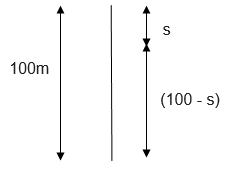For stone dropped from the tower,

s = ut + ½ gt2 = 0 + ½ (10)t2

s = 5t2…………(a)

For stone projected upward, s’ = ut – ½ gt2

(100 – s) = 25t – ½ 10t2

100 – s = 25t – 5t2……………(b)

From eq(a) we get

100 – 5t2 = 25t – 5t2

t = 4s

So stones will meet after 4s.

s = 5t2 = 5x(4)2 = 80m

So, the stones will meet at a distance of 80m from the top of the tower or 20m

(100 – 80)  from the base of the tower.

18. A ball thrown up vertically returns to the thrower after 6s, find

i. The velocity with which it was thrown up
ii. The maximum height it reaches
iii. Its position after 4s

Total time taken = 6s

Thus, time taken to reach the maximum height = 6/2 = 3s

From v = u – gt

0 = u – 9.8×3

u= 29.4m/s

The velocity with which the ball was thrown up is 29.4m/s.

From, v2 = u2 – 2gh

0 = (29.4)2 – 2×9.8xh

h = (29.4)2/ 2×9.8 = 44.1m

Maximum height attained by the ball is 44.1m/s

In first 3s the ball will rise and in the next 3s, it will fall back to the earth.

Therefore, Position after 4s = Distance covered in 1s of downward motion,

h = ut + ½ gt2 = 0 + 1/2 x 9.8x(1)2 = 4.9m

19. In what direction, does the buoyant force on an object immersed in a liquid act?

Buoyant force on an object immersed in a liquid acts in vertically upward direction.

20. Why does a block of plastic released under water come up to the surface?

The buoyant force acting on block of plastic by water is greater than the weight of the plastic block. So, plastic block when released under water, comes up to the surface

21. The volume of 50g of a substance is 20cm3. If density of water is 1g/cm3, will the substance float or sink?

Density of the substance, ρ = Mass/Volume = 50g/20cm3 = 2.5g/cm3

The density of the substance is greater than the density of water, so it will sink in water.

22. The volume of a 500g sealed packet is 350cm3. Will the packet float or sink in water, if the density of water is 1g/cm3? What will be the mass of the water displaced by this packet?

Density of the substance, ρ = Mass/Volume = 500/350 = 1.43g/cm3

The density of the substance is greater than the density of water, so it will sink in water.

Mass of water displaced by the packet= Volume of packet x density of water

= 350 x 1= 350g

### Topics Covered in Gravitation Class 9 Science

1. Gravitation
• UNIVERSAL LAW OF GRAVITATION
• IMPORTANCE OF THE UNIVERSAL LAW OF GRAVITATION
2. Free Fall
• TO CALCULATE THE VALUE OF g
• MOTION OF OBJECTS UNDER THE INFLUENCE OF GRAVITATIONAL FORCE OF THE EARTH
3. Mass
4. Weight
• WEIGHT OF AN OBJECT ON THE MOON
5. Thrust and Pressure
• PRESSURE IN FLUIDS
• BUOYANCY
• WHY OBJECTS FLOAT OR SINK WHEN PLACED ON THE SURFACE OF WATER?
6. Archimedes’ Principle
7. Relative Density